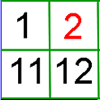#### You may also like### Prompt Cards

These two group activities use mathematical reasoning - one is numerical, one geometric.### Round and Round the Circle

What happens if you join every second point on this circle? How about every third point? Try with different steps and see if you can predict what will happen.### What's Left?

Use this grid to shade the numbers in the way described. Which numbers do you have left? Do you know what they are called?

# Dicey Array

##### Age 5 to 11Challenge Level

Thank you to everybody who sent in their ideas about this game. We had lots of different thoughts about which dice totals would be good to get or not so good to get - have a look at the ideas below and see which you agree with.

To start with, we had lots of solutions sent in from pupils at the ABQ Seeb International School in Oman. Jannah suggested that 12 would be a good total to get since there are many options, and 7 wouldn't be a good total to get since you would only have two choices.

Mohammed said:

The best dice totals are 18 and 20 and 12 because they have the most factors.

Sara said:

The best total to get is 12, 18 because you can make more factors (6).

The dice totals that are not so good to get are 2, 3, 5 and 7 because you get fewer factors (2).

Thank you all for sending in these ideas! The children from Burke Ward Primary School in NSW Australia sent in their wording of the rules and agreed that 12 and 18 were the best totals to get:

The best combinations to roll were 12 and 18 as they are the composite numbers that have the greatest total of combinations (arrays).

Ilham from St Aidan's Catholic Primary Academy in England said:

The best dice total to get is 6 + 6 = 12 since you can make 3 arrays and the worst dice totals include: 2, 3, 5, 7 and 11.

Well done, Ilham - if we are using two 0-9 dice, are there any other dice totals that would be just as bad as the ones you've found?

Most of the pupils who sent in their ideas said that it doesn't matter whether you go first or second because this is a game of chance. However, Taqiya from the ABQ Seeb International School in Oman thought that going first or second does make a difference. Why might it be easier to win if you go first?

Taqiya also had some ideas about how the game would change if you could add or subtract the numbers:

The way this changes the game is because in the game you have to add both the dice numbers and multiply but if you subtract a dice total then your number will decrease and you'll have a less likely chance to win.

Good idea, Taqiya. Why might having a smaller total make it less likely that you'll win?

Jannah thought that being able to add or subtract could be a good thing or a bad thing:

Adding and subtracting would give you more choices or would give you fewer choices. It depends on the number you get.

The children from Burke Ward Primary School found that if you can choose to either add or subtract, this means you have more chance of ending up with a helpful number:

We found that if you gave the person a chance to add or subtract the two dice values, it sometimes meant the difference between a prime or a composite number.

Good idea! Why are prime numbers particularly unhelpful in this game?

Ilham suggested one benefit of being able to subtract:

If you could choose to subtract you could make a more exact number (e.g. 5 - 3 = 2) and you might even step down into negative numbers!

That would certainly make the game very different! I wonder if we could still make arrays using negative numbers?

Naomi from the International School of Brussels in Belgium noticed something interesting about the number of arrays you can make for each dice total. She said:

Through the first game I realised you can only get even numbers because you're able to do the opposite, for example 4x2 and 2x4 (which are the same thing), but for the second game I realised for 3x3 there is no opposite because 3 and 3 are the same number so I got an odd number for how many possibilities there are.

This is very interesting, Naomi. It looks like having a dice total of 9 means you were able to make an odd number of arrays. I wonder which other dice totals would make an odd number of arrays?